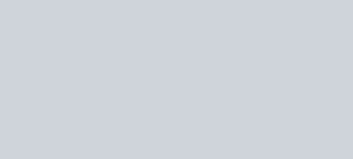Solve this following
Question:

If $A, B$ and $C$ are three sets such that $A \cap B=A \cap C$ and $A \cup B=A \cup C$, then :-

1. $B=C$

2. $A \cap B=\phi$

3. $A=B$

4. $A=C$

Correct Option: 1

Solution: